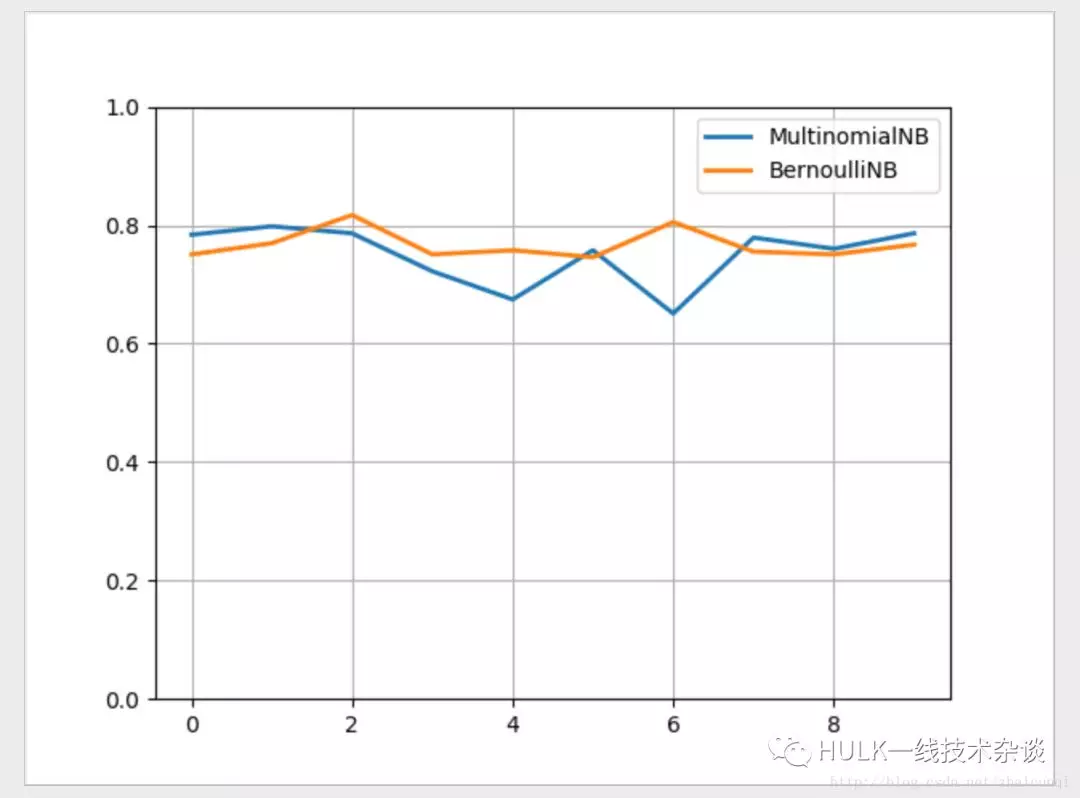# 一起学习朴素贝叶斯

2019 年 11 月 27 日## 算法原理

• 条件概率公式

• 全概率公式

• 特征条件独立假设

### 1 条件概率公式

P(A|B) = P(AB)/P(B)P(B|A) = P(AB)/P(A)所以：P(A|B) = P(B|A) * P(A) / P(B)

### 2 全概率公式

P(B) = P(A1B) + P(A1B) + ··· + P(AnB)     = ∑P(AiB)     = ∑P(B|Ai) * P(Ai) ·······················（i=1，2，····，n）

### 3 贝叶斯公式

P(Ak|B) = [ P(Ak) * P(B|Ak) ] / ∑P(B|Ai) * P(Ai） ·········（i=1，2，····，n）

P(Ak|B) = P(Ak) * P(B|Ak)

P(Ak) 先验概率，P(Ak|B) 后验概率，P(B|Ak) 似然函数

## 拉普拉斯平滑

P(y) = (|Dy| + 1) / (|D| + N)

P(xi|Dy) = (|Dy,xi| + 1) / (|Dy| + Ni)

## 文本分类

### 手动实现邮件分类

P(Spam|mail) = P(Spam) * ∏P(wi|Spam)

P(Normal|mail) = P(Normal) * ∏P(wi|Normal)

### 使用 sklearn 实现文本分类

# sklearn 实现文本分类import osimport random from numpy import *from numpy.ma import arangefrom sklearn.pipeline import Pipeline# TfidfVectorizer 文本特征提取（根据词出现的频率及在语句中的重要性）# HashingVectorizer 文本的特征哈希# CountVectorizer 将文本转换为每个词出现的个数的向量from sklearn.feature_extraction.text import TfidfVectorizerfrom sklearn.naive_bayes import BernoulliNBfrom sklearn.naive_bayes import MultinomialNBimport matplotlib.pyplot as plt# 获取样本集def get_dataset():    data = []    for root, dirs, files in os.walk(r'./mix20_rand700_tokens_cleaned/tokens/neg'):        for file in files:            realpath = os.path.join(root, file)            with open(realpath, errors='ignore') as f:                data.append((f.read(), 'bad'))    for root, dirs, files in os.walk(r'./mix20_rand700_tokens_cleaned/tokens/pos'):        for file in files:            realpath = os.path.join(root, file)            with open(realpath, errors='ignore') as f:                data.append((f.read(), 'good'))    random.shuffle(data)    return data    # 处理训练集与测试集def train_and_test_data(data_):    # 训练集和测试集的比例为7:3    filesize = int(0.7 * len(data_))    # 训练集    train_data_ = [each for each in data_[:filesize]]    train_target_ = [each for each in data_[:filesize]]    # 测试集    test_data_ = [each for each in data_[filesize:]]    test_target_ = [each for each in data_[filesize:]]    return train_data_, train_target_, test_data_, test_target_    """多项式模型：在多项式模型中， 设某文档d=(t1,t2,…,tk)，tk是该文档中出现过的单词，允许重复，则先验概率P(c)= 类c下单词总数/整个训练样本的单词总数类条件概率P(tk|c)=(类c下单词tk在各个文档中出现过的次数之和+1)/(类c下单词总数+|V|)V是训练样本的单词表（即抽取单词，单词出现多次，只算一个），|V|则表示训练样本包含多少种单词。 P(tk|c)可以看作是单词tk在证明d属于类c上提供了多大的证据，而P(c)则可以认为是类别c在整体上占多大比例(有多大可能性)。"""def mnb(train_da, train_tar, test_da, test_tar):    nbc = Pipeline([        ('vect', TfidfVectorizer()),        ('clf', MultinomialNB(alpha=1.0)),    ])    nbc.fit(train_da, train_tar)  # 训练我们的多项式模型贝叶斯分类器    predict = nbc.predict(test_da)  # 在测试集上预测结果    count = 0  # 统计预测正确的结果个数    for left, right in zip(predict, test_tar):        if left == right:            count += 1    # print("多项式模型：", count / len(test_target))    return count / len(test_tar)"""伯努利模型：P(c)= 类c下文件总数/整个训练样本的文件总数P(tk|c)=(类c下包含单词tk的文件数+1)/(类c下单词总数+2)"""def bnb(train_da, train_tar, test_da, test_tar):    nbc_1 = Pipeline([        ('vect', TfidfVectorizer()),        ('clf', BernoulliNB(alpha=1.0)),    ])    nbc_1.fit(train_da, train_tar)  # 训练我们的多项式模型贝叶斯分类器    predict = nbc_1.predict(test_da)  # 在测试集上预测结果    count = 0  # 统计预测正确的结果个数    for left, right in zip(predict, test_tar):        if left == right:            count += 1    # print("伯努利模型：", count / len(test_target))    return count / len(test_tar)# 训练十次x = arange(10)y1 = []y2 = []for i in x:    print(i)    data = get_dataset()    train_data, train_target, test_data, test_target = train_and_test_data(data)    y1.append(mnb(train_data, train_target, test_data, test_target))    y2.append(bnb(train_data, train_target, test_data, test_target))print(x)print(y1)print(y2)plt.plot(x, y1, lw='2', label='MultinomialNB')plt.plot(x, y2, lw='2', label='BernoulliNB')plt.legend(loc="upper right")plt.ylim(0, 1)plt.grid(True)plt.show()

sklearn 结果对比## 总结

Scikit learn 也简称 sklearn, 是机器学习领域当中最知名的 python 模块之一。Sklearn 把所有机器学习的模式整合统一起来了，学会了一个模式就可以通吃其他不同类型的学习模式。

2019 年 11 月 27 日 10:29268

## 评论

• ###### 建模非正态分布：广义线性模型

今天我来和你分享广义线性模型的概念与原理，它克服了狭义线性模型的一些限制，拓展了线性模型的应用范围。

2018 年 7 月 10 日

• ###### 向量空间模型：如何让计算机理解现实事物之间的关系？

向量空间模型充分利用了空间中向量的距离和夹角特性，来描述文档和查询之间的相似程度，或者说相关性。

2019 年 3 月 4 日

• ###### 吴恩达团队提出倒计时回归模型：用 AI 技术预测病患死亡时间

近日，斯坦福大学Andrew Ng团队开发出了一种使用人工智能来预测病人死亡时间的系统。科学家希望该系统可以为病人提供更好的临床关怀。作者提出了一种新的预报检验方法Survival-CRPS，通过优化连续分级概率评分来提高预报的锐度，同时保持预报的校准度。这是科学界首次将最大似然法之外的评分方法成功应用于大型生存预测任务。

• ###### 一文看懂 AutoEncoder 模型演进图谱

本文主要介绍AutoEncoder系列模型框架的演进，旨在梳理AutoEncoder的基本原理。

• ###### 最简单的概率图：朴素贝叶斯

从今天起，我们将进入概率图模型的模块，以贝叶斯的角度重新审视机器学习。

2018 年 8 月 9 日

• ###### 26 丨 K-Means（上）：如何给 20 支亚洲球队做聚类？

假设我有20支亚洲足球队，想要将它们按照成绩划分成3个等级，可以怎样划分？

2019 年 2 月 11 日

• ###### 多标签图像分类综述

图像分类作为计算机视觉领域的基础任务，经过大量的研究与试验，已经取得了傲人的成绩。然而，现有的分类任务大多是以单标签分类展开研究的。当图片中有多个标签时，又该如何进行分类呢？

• ###### 机器学习在高德地图轨迹分类的探索和应用

本文从给予概率密度分布的贝叶斯模型视角与基于轨迹点图像编码的深度学习视角分别探索了轨迹分类可能的技术方案。

• ###### 如何使用半监督学习为结构化数据训练出更好的深度学习模型

本文将使用半监督学习来提高深度神经模型在低数据环境下应用于结构化数据时的性能。

• ###### 08 机器学习 | 简约而不简单：线性回归

数学中的线性模型可谓“简约而不简单”：它既能体现出重要的基本思想，又能构造出功能更加强大的非线性模型。

2017 年 12 月 26 日

• ###### Leslie G. Valiant 获得 2010 年图灵奖

因其在计算学习理论及广泛的计算机科学领域里作出的重大贡献，Leslie G. Valiant获得2010年ACM图灵奖。

• ###### 基于 AFK-MC²算法的 k-Means 聚类加速算法

Olivier Bachem等人在其NIPS 2016（Neural Information Processing Systems，神经信息处理系统大会，机器学习领域的顶级会议之一）的文章“Fast and Probably Good Seedings for k-Means”中提出了AFK-MC²算法，该算法改进了k-Means算法中初始种子点的生成方式，使其聚类速度相较于目前最好的k-Means++方式提高了好几个数量级。

• ###### 文本数据的机器学习自动分类方法 (上)

以统计理论为基础，利用机器学习算法对已知的训练数据做统计分析从而获得规律，再运用规律对未知数据做预测分析，已成为文本分类领域的主流。InfoQ联合“达观数据“共同策划了《文本数据的机器学习自动分类方法》系列文章，为您详细阐述机器学习文本分类的基本方法与处理流程。

• ###### 频率视角下的机器学习

频率主义解决统计问题的基本思路如下：参数是确定的，数据是随机的，利用随机的数据推断确定的参数，得到的结果也是随机的。

2018 年 6 月 5 日

• ###### 频率视角下的机器学习

《机器学习40讲》，3个月帮你打通机器学习的任督二脉。

6 月日更

#### 我的程序员生涯(2)Leader修炼指“北”：管理路上的大小Boss

Leader修炼指“北”：管理路上的大小Boss